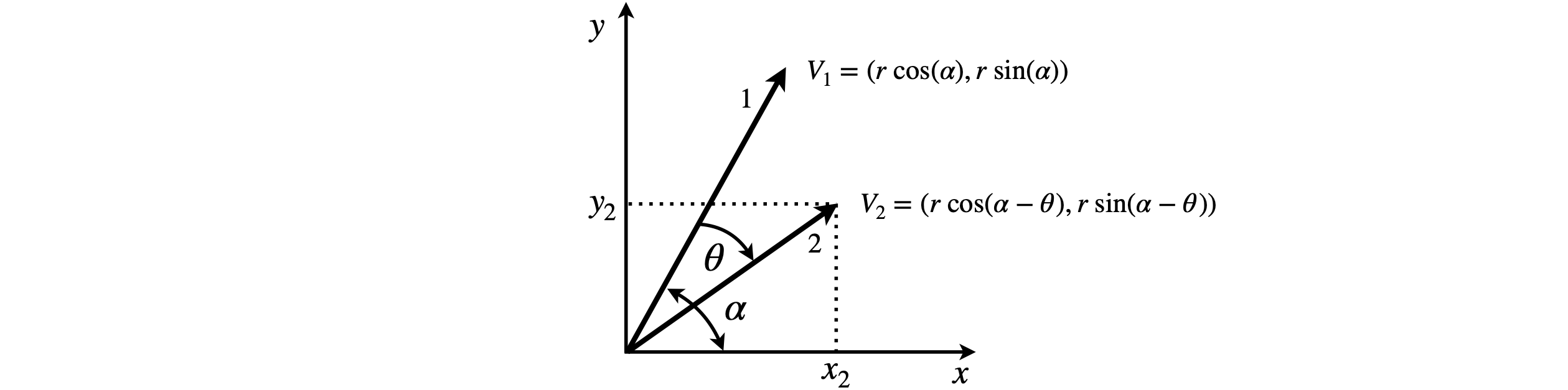# Questions 31 - 34#

## Q31#

Derive the equations 10 and matrix 11 for rotating the tip of a vector clockwise using the definitions in Fig. 34. The two vectors defined in terms of their polar coordinates, are $$(r, \cos(90 -\alpha)$$ and $$(r, \cos(90 -\alpha + \theta))$$ and their Cartesian equivalents, $$V_1$$ and $$V_2$$.Figure 34. Rotating vectors

Strategy: Define the $$x$$ and $$y$$ coordinates of both vectors in terms of sines and cosines. Expand the double-angle sine and cosine produced using the trig formulas.

## Q32 Rotation#

Show that by rotating a molecule by $$\theta$$ using the rotation matrix $$R(\theta)$$, equation 11, and then again by $$\theta$$, the result is the same as the operation $$R(\theta)^2$$.

Strategy: The total angle moved is $$2\theta$$, so we need to show that the rotation matrix $$\pmb{R}$$ with angle $$2\theta$$ is the same as $$\pmb{R}^2$$ with angle $$\theta$$ so we are likely to need the double-angle trig formulas which are $$\sin(2\theta) = 2 \sin(\theta)\cos(\theta)$$ and $$\cos(2\theta) = \cos^2(\theta) - \sin^2(\theta)$$.

## Q33 Rotate a molecule#

Draw and rotate part of a protein structure you choose; this could be based on algorithm 4. Use part of the peptide chain from a .pdb file taken from the Brookhaven database. The size is not so important and will be limited by how easily you can make a connectivity list. Alternatively, you could try to generate this list by measuring the distance between atoms using known bond lengths. These can also be obtained from the coordinates in the .pdb file.

## Q34 CCl$$_4$$#

The molecule CCl$$_4$$ has tetrahedral symmetry where the bond angles are each $$109.7^\text{o}$$. The atoms labelled 3 and 4 are in the $$z-y$$ plane and the other two in the $$z-x$$ plane.

(a) Using a matrix representation for each symmetry operation, show that a rotation by $$90^\text{o}$$ about the z-axis, then inversion followed by an $$S_4$$ rotation-reflection operation, leaves the molecule in an indistinguishable state.

(b) Does operating in the reverse order produce the same result?Figure 35. Carbon tetrachloride

Strategy: If the angle rotated about the z-axis is $$\theta$$ the rotation matrix when $$\theta = 90^\text{o}$$ contains elements of zero and $$\pm 1$$ only if the other rotation angles are zero. Inversion inverts all the atoms coordinates.